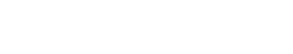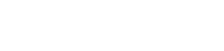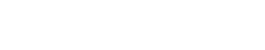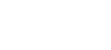# Implement Bezier Curve using C# in UnityIn this tutorial, we will learn how to implement the Bezier curve using C# in Unity. We will then create a sample application that displays the Bezier curve.

This tutorial is the first part of the tutorial from a larger tutorial on Creating a Jigsaw Puzzle Game in Unity.

Find the GitHub repository of this tutorial at https://github.com/shamim-akhtar/jigsaw-puzzle.Click to Play the WebGL version on your browser (mobile devices may not be able to play)

Contact me

## Bezier Curve

A Bézier curve is a parametric curve defined by a set of points known as control points. It is widely used in computer graphics and related fields. For a more detailed understanding of Bezier curves, refer to the Wikipedia page.

The generic definition of a point in the Bezier curve isWhere n is the degree of the curve andare the Binomial coefficients. We can represent it as below.We can simplify the main equation at the top towhereare known as Bernstein basis polynomials of degree n.

For an n degree curve, there will be + 1 control point. When the number of control points is two (or a degree of one; n = 1), a Bezier curve becomes a straight line and is equivalent to linear interpolation. When the number of control points is three (or a degree of 2; n = 2), a Bezier curve becomes a parabola.

Our first task will be to implement a bezier curve given a set of control points. You can find a lot of tutorials online on how to create a Bezier curve. However, for the sake of this tutorial, we will make our implementation of the Bezier curve in C#.

Please create a new Unity2D project and name it Bezier. Please create a new folder in the Assets directory and call it Scripts.

Right-click on the Scripts folder in the Unity Editor’s Project window and create a new C# script. Name it BezierCurve. Double-click and open it in Visual Studio or your favourite IDE. Remove Monobehavior (we do not want this class to derive from Monobehavior), the Start and the Update methods. The class should look like below.

``````using System.Collections;
using System.Collections.Generic;
using UnityEngine;

public class BezierCurve
{
}
```Code language: C# (cs)```

Now, let’s see what needs to be there in this class. At the least, we will require a function that returns an interpolated Bezier point given t, where t can be between 0 and 1 (both inclusive), and a list of control points. This function will correspond to the equation:

We can see that the inputs to the function are t and the list of control points. Go ahead and create the procedure that will return us a Bezier point given these two inputs.

``````public class BezierCurve
{
public static Vector3 Point3(float t, List<Vector3> controlPoints)
{
}
}
```Code language: C# (cs)```

Note that we made the function static so that we do not have to instantiate a BezierCurve class to get the Bezeir points given a set of control points. This function should be self-sustainable and should do our job of calculating the Bezier point.

We now want to implement the actual calculation of the Bezier point. To do so, let’s analyze and break down the function below.whereandIf we look from below to up, we will find that the three diagrams seem not too difficult to implement. Let’s start from the bottom. Calculate the Binomial coefficient with inputs n and i; both integer values.To do so, we will have to calculate the values of

• The Factorial of n,
• The Factorial of i, and
• The Factorial of (n – i).

We can implement a function called Factorial that calculates the factorial value given an integer input. Even better, we can precalculate the Factorial values of numbers up to a maximum value of n (let’s say 16 or 20) and use that. So for any Bezier curve that has a degree of this maximum value or less will be able to create the Bezier curve. Now, we can go beyond this maximum value and make a generic factorial function, but this value will suffice for our job for now.

``````public class BezierCurve
{
// a look up table for factorials. Capped to 16.
private static float[] Factorial = new float[]
{
1.0f,
1.0f,
2.0f,
6.0f,
24.0f,
120.0f,
720.0f,
5040.0f,
40320.0f,
362880.0f,
3628800.0f,
39916800.0f,
479001600.0f,
6227020800.0f,
87178291200.0f,
1307674368000.0f,
20922789888000.0f,
};
****
}
```Code language: C# (cs)```

With this lookup table, we are okay to proceed with the implementation of the Binomial coefficient.

``````private static float Binomial(int n, int i)
{
float ni;
float a1 = Factorial[n];
float a2 = Factorial[i];
float a3 = Factorial[n - i];
ni = a1 / (a2 * a3);
return ni;
}
```Code language: C# (cs)```

Note that the function is private as we will only allow internal access to this function. We did not do a validation check if n <= 16. We want the caller of this function to ensure that n <= 16.

Next, we will calculate the Bernstein basis polynomials shown by the following equation.To do so, we will need to calculate the Binomial coefficient and the two power terms. We have already calculated the Binomial coefficient above. The below function shows the implementation of the calculation of Bernstein basis polynomials.

``````private static float Bernstein(int n, int i, float t)
{
float t_i = Mathf.Pow(t, i);
float t_n_minus_i = Mathf.Pow((1 - t), (n - i));

float basis = Binomial(n, i) * t_i * t_n_minus_i;
return basis;
}
```Code language: C# (cs)```

Finally, we calculate the Bezier point as given by the equation below.This calculation is just the summation of the Bernstein basis polynomials for all the control points. We can implement it as follows.

``````public static Vector3 Point3(float t, List<Vector3> controlPoints)
{
int N = controlPoints.Count - 1;
if (N > 16)
{
Debug.Log("You have used more than 16 control points. The maximum control points allowed is 16.");
controlPoints.RemoveRange(16, controlPoints.Count - 16);
}
if (t <= 0) return controlPoints;
if (t >= 1) return controlPoints[controlPoints.Count - 1];

Vector3 p = new Vector3();

for (int i = 0; i < controlPoints.Count; ++i)
{
Vector3 bn = Bernstein(N, i, t) * controlPoints[i];
p += bn;
}

return p;
}
```Code language: C# (cs)```

We have successfully implemented the calculation of a Bezier point for a given set of control points at an interval t.

If we want to get a whole list of Bezier points that represents the Bezier curve, then we will need to calculate the Bezier points for the entire duration starting from t = 0 (where the point is the same at the start control point) to t = 1 (where the point is the same as the end control point). The interval duration will depend on the nature of the problem and the distance of the points. For simplicity, we can use 0.01 as the interval. Choosing 0.01 as an interval will mean that there will be 100 points in the Bezier curve.

Let’s implement the method that returns the list of points representing the Bezier curve.

``````public static List<Vector3> PointList3(
List<Vector3> controlPoints,
float interval = 0.01f)
{
int N = controlPoints.Count - 1;
if (N > 16)
{
Debug.Log("You have used more than 16 control points. " +
"The maximum control points allowed is 16.");
controlPoints.RemoveRange(16, controlPoints.Count - 16);
}

List<Vector3> points = new List<Vector3>();
for (float t = 0.0f; t <= 1.0f + interval - 0.0001f; t += interval)
{
Vector3 p = new Vector3();
for (int i = 0; i < controlPoints.Count; ++i)
{
Vector3 bn = Bernstein(N, i, t) * controlPoints[i];
p += bn;
}
}

return points;
}
```Code language: C# (cs)```

Similarly, we can implement the same for Vector2, as shown below.

``````public static Vector2 Point2(float t, List<Vector2> controlPoints)
{
int N = controlPoints.Count - 1;
if (N > 16)
{
Debug.Log("You have used more than 16 control points. The maximum control points allowed is 16.");
controlPoints.RemoveRange(16, controlPoints.Count - 16);
}

if (t <= 0) return controlPoints;
if (t >= 1) return controlPoints[controlPoints.Count - 1];

Vector2 p = new Vector2();

for (int i = 0; i < controlPoints.Count; ++i)
{
Vector2 bn = Bernstein(N, i, t) * controlPoints[i];
p += bn;
}

return p;
}
public static List<Vector2> PointList2(
List<Vector2> controlPoints,
float interval = 0.01f)
{
int N = controlPoints.Count - 1;
if (N > 16)
{
Debug.Log("You have used more than 16 control points. " +
"The maximum control points allowed is 16.");
controlPoints.RemoveRange(16, controlPoints.Count - 16);
}

List<Vector2> points = new List<Vector2>();
for (float t = 0.0f; t <= 1.0f + interval - 0.0001f; t += interval)
{
Vector2 p = new Vector2();
for (int i = 0; i < controlPoints.Count; ++i)
{
Vector2 bn = Bernstein(N, i, t) * controlPoints[i];
p += bn;
}
}

return points;
}
```Code language: C# (cs)```

We have concluded our implementation of the BezierCurve class.

## Testing Bezier Curves

Rename the scene to BezierTest. We want to create a display for our control points. In the next section, we will create a prefab that we will use for displaying our control points on the scene.

### Point Prefab

Right-click and add a new Circle Sprite.

We will use this sprite to represent our control points. Rename the sprite to Point.

Select this sprite and click on Add Component from the Inspector. Select New Script and name it Point_Viz. Double-click Point_Viz script and open in Visual Studio or your favourite IDE.

We want this sprite to be able to select and drag using our mouse. Go ahead and add the following code into your Point_Viz.cs.

Add a private variable named mOffset.

Add OnMouseDown, OnMouseDrag and OnMouseUp methods and implement the necessary code to use mouse click to select the sprite and drag to move it.

``````void OnMouseDown()
{
if (EventSystem.current.IsPointerOverGameObject())
{
return;
}

mOffset = transform.position - Camera.main.ScreenToWorldPoint(
new Vector3(Input.mousePosition.x, Input.mousePosition.y, 0.0f));

}

void OnMouseDrag()
{
if (EventSystem.current.IsPointerOverGameObject())
{
return;
}
Vector3 curScreenPoint = new Vector3(
Input.mousePosition.x,
Input.mousePosition.y, 0.0f);
Vector3 curPosition = Camera.main.ScreenToWorldPoint(curScreenPoint) + mOffset;
transform.position = curPosition;
}
void OnMouseUp()
{
if (EventSystem.current.IsPointerOverGameObject())
{
return;
}

}
```Code language: C# (cs)```

To select this sprite, we will also need to add a Collider to the sprite. Select this sprite and add a new component called Circle Collider 2D.

Create a new folder called Resources in Assets. Now create a new folder called Prefabs in the Resources folder.

Drag and drop this Point sprite into the Prefabs folder and name it PointPrefab and then remove Point from the scene.

### Bezier Curve Visualization

Right-click on the Hierarchy window and add a new Empty GameObject.

Rename this empty game object to Bezier_Viz. We will use this game object to display our Control Points and the Bezier curve.

Select Bezier_Viz and add a new script component called Bezier_Viz. Double-click on Bezier_Viz script and open in Visual Studio or your favourite IDE.

Add the following variables to Bezier_Viz.cs file.

First of all, we add a public variable of type List<Vector2> called ControlPoints. This will hold the first few control points that we set from the Unity Editor.

Then we will use a public variable that will hold the PointPrefab. We will create instances of this prefab to display our control points.

We will use LineRenderer to show our lines and curves. We want to have two LineRenderer; the first one is to show straight lines connecting the various control points, and the second one is to show the Bezier curve.

Then we want to have a List<GameObject> to hold our instantiated control point game objects.

The final few parameters are for decorative purposes. We will allow setting the line width and line colour from the Unity editor.

We are now all set to implement the necessary functionality.

``````private LineRenderer CreateLine()
{
GameObject obj = new GameObject();
lr.startColor = LineColour;
lr.endColor = LineColour;
lr.startWidth = LineWidth;
lr.endWidth = LineWidth;
return lr;
}
```Code language: C# (cs)```

In the above code, we create the default LineRenderer.

Then in the Start method, we, first of all, create the LineRenderers and then the control points.

``````void Start()
{
// Create the two LineRenderers.
mLineRenderers = new LineRenderer;
mLineRenderers = CreateLine();
mLineRenderers = CreateLine();

// set a name to the game objects for the LineRenderers
// to distingush them.
mLineRenderers.gameObject.name = "LineRenderer_obj_0";
mLineRenderers.gameObject.name = "LineRenderer_obj_1";

// Create the instances of PointPrefab
// to show the control points.
for (int i = 0; i < ControlPoints.Count; ++i)
{
GameObject obj = Instantiate(PointPrefab,
ControlPoints[i],
Quaternion.identity);
obj.name = "ControlPoint_" + i.ToString();
}
}

```Code language: C# (cs)```

Finally, in the Update method, we set values to the mLineRenderer and mLineRenderer.

``````// Update is called once per frame
void Update()
{
LineRenderer lineRenderer = mLineRenderers;
LineRenderer curveRenderer = mLineRenderers;

List<Vector2> pts = new List<Vector2>();

for (int k = 0; k < mPointGameObjects.Count; ++k)
{
}

// create a line renderer for showing the straight
//lines between control points.
lineRenderer.positionCount = pts.Count;
for (int i = 0; i < pts.Count; ++i)
{
lineRenderer.SetPosition(i, pts[i]);
}

// we take the control points from the list of points in the scene.
// recalculate points every frame.
List<Vector2> curve = BezierCurve.PointList2(pts, 0.01f);
curveRenderer.startColor = BezierCurveColour;
curveRenderer.endColor = BezierCurveColour;
curveRenderer.positionCount = curve.Count;
for (int i = 0; i < curve.Count; ++i)
{
curveRenderer.SetPosition(i, curve[i]);
}
}

```Code language: C# (cs)```

In the first section of the Update method, we get the position of the control points from the instantiated game objects for points. This is because we may change the position of the control points by clicking on any one of them and relocate.

In the code below, we are accumulating the points from the list of the game instantiated game objects that represent the control points.

``````for (int k = 0; k < mPointGameObjects.Count; ++k)
{
}
```Code language: C# (cs)```

Then we are setting these points to the first LineRenderer.

``````lineRenderer.positionCount = pts.Count;
for (int i = 0; i < pts.Count; ++i)
{
lineRenderer.SetPosition(i, pts[i]);
}
```Code language: C# (cs)```

In the second section, we get the Bezier points by calling the static BezierCurve.PointList2 method. This method returns a list of Vector2. Note that we have used the interval to be 0.01. So we will get 101 points on our list. We then set these points to our second LineRenderer. We also make the colour to be the one we set for the Bezier curve.

``````List<Vector2> curve = BezierCurve.PointList2(pts, 0.01f);
curveRenderer.startColor = BezierCurveColour;
curveRenderer.endColor = BezierCurveColour;
curveRenderer.positionCount = curve.Count;
for (int i = 0; i < curve.Count; ++i)
{
curveRenderer.SetPosition(i, curve[i]);
}
```Code language: C# (cs)```

Now we are all set. Before we run the program, we will need to do some settings in our Unity editor.

### Setting Values in Unity Editor

Go to your Unity editor. Select the Bezier_Viz game object from the hierarchy. Drag and drop the PointPrefab to the Point Prefab field of the Bezier_Viz script component (shown below).

Also, add an EventSystem so that we can capture mouse inputs.

Create 3 control points as shown below. Set the values for each of these control points. Set the Line Width to be 0.05.

Click play. You should see it as below.

## Enhancements

In the following section, we will implement one new functionality. This functionality is to allow adding new control points. We will add new control points by double-clicking on the screen (up to a maximum number of 16).

Open Bezier_Viz.cs file and add the following code

``````void OnGUI()
{
Event e = Event.current;
if (e.isMouse)
{
if (e.clickCount == 2 && e.button == 0)
{
Vector2 rayPos = new Vector2(
Camera.main.ScreenToWorldPoint(Input.mousePosition).x,
Camera.main.ScreenToWorldPoint(Input.mousePosition).y);

InsertNewControlPoint(rayPos);
}
}
}

void InsertNewControlPoint(Vector2 p)
{
if (mPointGameObjects.Count >= 16)
{
Debug.Log("Cannot create any new control points. Max number is 16");
return;
}
GameObject obj = Instantiate(PointPrefab, p, Quaternion.identity);
obj.name = "ControlPoint_" + mPointGameObjects.Count.ToString();
}

```Code language: C# (cs)```

Run the program by clicking on the Play button in Unity. Double-click to add new control points, as shown below.Testing Bezier curves in Unity – Bezier Curve with new control points.

We have completed Part 1 of the tutorial. In the next section, Part 2 – Create a Jigsaw Tile from an Existing Image, we will learn how to create a Jigsaw tile from an existing image using the Bezier curve.

Playe the WebGL version of the Jigsaw Game.

1.2.